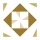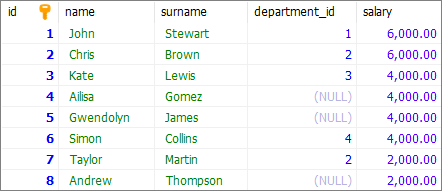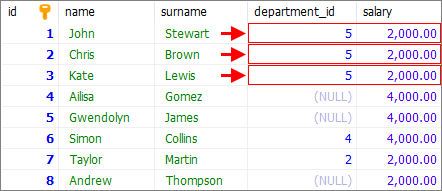Home
IT Knowledge
Inspiration
Languages
EN

# MS SQL Server - Update multiple rows at once

1 points
Created by:Suzannah-Estrada
340

In this article, we would like to show you how to update multiple rows at once in MS SQL Server.

Quick solution:

``````UPDATE [table_name]
SET [column1] = value1, [column2] = value2, [columnN] = valueN
WHERE condition;``````

## Practical example

To show you how to update multiple rows at once, we will use the following `users` table:MS SQL Server - example data used to count rows with NULL values

Note:

At the end of this article you can find database preparation SQL queries.

### Example

In this example, we will update `department_id` and `salary` value of the first three users from the `users` table.

Query

``````UPDATE [users]
SET [department_id] = 5, [salary] = 6000
WHERE [id] <= 3;``````

or

``````UPDATE [users[SET [department_id] = 5, [salary] = 6000 WHERE [id] = 1;
UPDATE [users[SET [department_id] = 5, [salary] = 6000 WHERE [id] = 2;
UPDATE [users[SET [department_id] = 5, [salary] = 6000 WHERE [id] = 3;``````

Result:MS SQL Server - update multiple rows at once - result

## Database preparation

`create_tables.sql` file:

``````CREATE TABLE [users] (
[id] INT IDENTITY(1,1),
[name] VARCHAR(50) NOT NULL,
[surname] VARCHAR(50) NOT NULL,
[department_id] INT,
[salary] DECIMAL(15,2) NOT NULL,
PRIMARY KEY ([id])
);``````

`insert_data.sql` file:

``````INSERT INTO [users]
( [name], [surname], [department_id], [salary])
VALUES
('John', 'Stewart', 1, '6000'),
('Chris', 'Brown', 2, '6000'),
('Kate', 'Lewis', 3, '4000'),
('Ailisa', 'Gomez', NULL, '4000'),
('Gwendolyn', 'James', NULL, '4000'),
('Simon', 'Collins', 4, '4000'),
('Taylor', 'Martin', 2, '2000'),
('Andrew', 'Thompson', NULL, '2000');``````